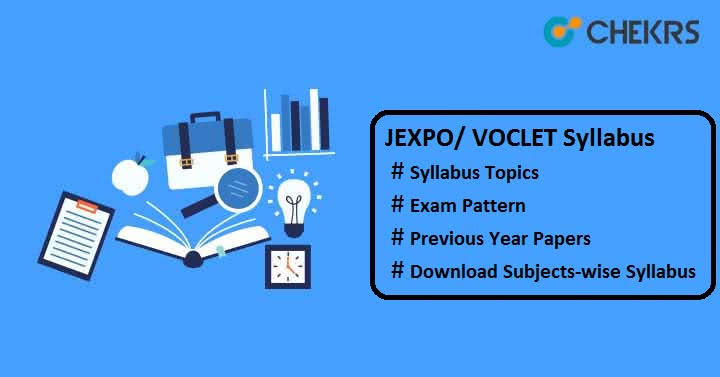JEXPO/ VOCLET Syllabus 2020 Pdf Download: West Bengal State Council of Technical Education (WBSCTE) conducts entrance test every year to provide admission into polytechnic courses. To get admission in the first year of Diploma courses in the field of engineering & technology, candidates need to check the complete WB JEXPO Syllabus along with the JEXPO Exam Pattern.The syllabus is comprised of class 10th Mathematics & Science subjects. Here we have provided the complete topic-wise syllabus, go through it and start preparing for the exam.

SubjectsTopics
Mathematics
• Real Numbers
• Laws of Indices
• Graph
• Co-ordinate Geometry – Distance Formula
• Linear Simultaneous Equations
• Transversal & Mid-Point Theorem
• Profit & Loss
• Statistics
• Theorems on Area
• Construction of a Parallelogram whose measurement of one angle is given and equal in area of a Triangle
• Construction of a Triangle equal in area of a quadrilateral, Properties of Parallelogram
• Polynomial
• Co-ordinate Geometry – Internal and External Division of Straight Line Segment
• Factorization
• Area & Perimeter of Triangle & Quadrilateral shaped region
• Circumference of Circle
• Co-ordinate Geometry – Area of Triangular Region
• Logarithm
• Theorems on concurrence
• Area of circular region
• Quadratic Equations with one variable
• Simple Interest
• Ratio and Proportion
• Compound Interest and Uniform Rate of Increase or Decrease
• Theorems related to circle
• Rectangular Parallelopiped or Cuboids
• Theorems related to Angles in a Circle
• Right Circular Cylinder
• Theorems related to Tangent to a Circle
• Right Circular Cone
• Similarity-Construction of tangent to a circle
• Theorems related to Cyclic Quadrilateral
• Construction of circumcircle and incircle of a triangle
• Sphere
• Variation
• Real life Problems related to different Solid Objects
• Trigonometry- Concept of Measurement of Angle
• Construction – Determination of Mean Proportional
• Trigonometric Ratios of Complementary angle
• Application of Trigonometric Ratios – Heights & Distances
• Statistics –Mean, Median, Ogive, Mode and Numerical Aptitude
• Pythagoras Theorem
• Trigonometric Ratios and Trigonometric Identities.
Physics & Chemistry
• Measurement
• Force & Motion
• Solution
• Acids, Bases & Salts
• Atomic Structure
• Mole Concept
• Work, Power & Energy
• Sound
• Heat
• Matter – Structure and Properties
• Separation of Components of Mixtures
• Water
• Behavior of Gases
• Light
• Ionic and Covalent Bonding
• Chemical Calculations
• Thermal Phenomena
• Current Electricity
• Periodic Table
• Periodicity of the Properties of Elements
• Electricity and Chemical Reactions
• Metallurgy
• Atomic Nucleus
• Organic Chemistry
• General Awareness
• Inorganic Chemistry

Aspirants preparing for VOLCET-2020 must check the given VOLCET Syllabus including important topics. The syllabus is prescribed as per the WB State Council of Technical and Vocational Education and Skill Development (Vocational Education Division).

### VOCLET Syllabus 2020.

SubjectsTopics
Mathematics
• Law of Indices, Complex Numbers
• Sequence & Series: AP, GP
• Binomial Theorem
• Matrix, Determinant
• Trigonometric
• Co-ordinate System
• Calculus
Physics
• Units of Measurements
• Work, Energy & Power
• Scalar & Vector quantities
• Center of Mass
• Law of Gravitation
• Elastic Behaviour
• Thermal Physics
• Periodic Motion
Chemistry
• Chemical Equation
• Extra nuclear Structure of Atom
• Chemical Bonding & Molecular structure
• State of Matter: Gaseous & Liquid
• Thermodynamics System
• Equilibrium and Acidimetry
• Organic Chemistry & Organic Compounds
• Environmental Chemistry
Computer Application
• Introduction to Computer Systems
• Computer Software & Programming Languages
• DOS: Working with MS DOS commands
• Windows OS
• Word Processing
• Internet Browsing, Search Engines uses, E-mail
• Numerical Aptitude
Fundamentals of Mechanics
• Concept of Engineering Mechanics
• System of Forces
• Moment & its Application
• Equilibrium of Force System
• Friction
• Simple Lifting Machines
• Center of Gravity
• Moment of Inertia
• General Awareness

### JEXPO 2020 Exam Pattern

Applicants must know the Exam Pattern before appearing for the exam, as it guides how to attempt the paper and score good marks in examination.

 Mode of Exam Offline Mode Duration of Exam 2 hours for each paper Paper Language English Type of Questions Multiple Choice Type Questions Total Questions 200 Papers Subjects No of Questions Marks per Question Total Marks Paper-1 Mathematics 100 1 100 Paper-2 Physics 50 50 Chemistry 50 50 Marking Scheme 1 mark will be given for each correct answer. No negative marking.

#### JEXPO Previous Year Papers

Candidates who have completed the entire syllabus need to check the JEXPO Previous Year Papers to get rough idea of original papers. For better preparation, you should give Online Mock Test on a regular basis. Solving Sample Papers will help you to improve your speed and accuracy and you will learn how to manage the time. Here we have provided the Question Paper Download link to help those candidates who are looking for the previous year question papers.

Still, you have any query related to  WB Polytechnic Syllabus and exam pattern or previous year question papers, you can write your question in the below comment section and wait for our response. Good Luck!!!